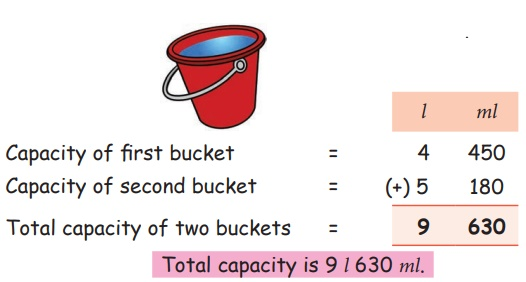Home | | Maths 4th Std | Subtraction using standard units (litre and ml)

# Subtraction using standard units (litre and ml)

Subtraction using standard units (litre and ml)

Subtraction using standard units (litre and ml)

EXAMPLE 1

Subract 8 l 450 ml from 28 l 750 ml.Step 1: Subtract 450 ml from 750 ml

Step 2: Subtract 8 l from 28 l

Therefore 28 l 750 ml − 8 l 450 ml = 20 l 300 ml

Life related problems

EXAMPLE 2

Sharma family have 2 buckets in their house.

The capacity of one bucket is 4 l 450 ml and the other one is 5 l 180 ml. What is the total capacity of two buckets?Total capacity is 9 l 630 ml.

EXAMPLE 3

A motor car consumed 188 l of petrol and 145 l 375 ml in the Month of January and February. What is the difference in the petrol consumption?